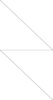# mining

 Name mining Download mining.mps.gz Set Membership Challenge Problem Status Open Problem Feasibility Feasible Originator/Contributor K. Eurek Rows 661133 Cols 348921 Num. non-zeros in A 3844879 Num. non-zeros in c 348921 Rows/Cols 1.8947928041 Integers Binaries 348920 Continuous 1 min nonzero |Aij| 0.6859025 max |Aij| 27044.12 min nonzero |cj| 0.03020538 max |cj| 948653700 Integer Objective Unknown LP Objective -949724584.696851 Aggregation Variable Bound 347953 Set partitioning Set packing Set covering Cardinality Equality Knapsacks Bin packing Invariant Knapsack 313101 Knapsacks Integer Knapsack Mixed 0/1 78 General Cons. References

Unspecified mining application.

Mark Zuckerberg suggests the following tightening of one class of constraints:
The variables in this problem (except for the last one, x348921, which is fixed to 1 and does not appear in any constraint) are arranged in groups of 20, and there are three classes of precedence constraints (or more accurately, implication constraints):
1) For each variable index i=...,348920, if i mod 20 > 0 then there is a constraint x_i <= x_{i+1} (so x1 <= x2 <= ... <= x20, x21<=x22 <= ... <= x40, etc).
2) If i mod 20 = and i>20 then there is a constraint x_i <= x_{i-20}, (so x1 >= x21 >= x41 >=...)
3) and for all other i there is a constraint x_i <=_{i-1} + x_{i-20} (so x22 <= x21 + x2, x23 <= x22 + x3,..., x40 <= x39 + x20, x42 <= x41 + x22,..., x60 <= x59 + x40,...).
Graphically, we can represent the variables as a table with height twenty and width 348920 / 20, with indices 1,...,20 going down the left-most column, then 21,...,40 going down the next column to the right, etc. There is then a precedence constraint (1) from each variable in a column to the one below it, a precedence constraint (2) from the topmost entry in each column to its neighbor on the left, and an "OR" precedence constraint (3) from each entry that isn't on top of a column, requiring that if it has value 1 then either the one above it or the one to its left must have value 1 as well.
But in fact this OR precedence (3) can be replaced with a simple precedence constraint from each variable to the one at its left. To see this note that in any column, the first type of precedence constraint will require that a feasible solution must be a contiguous sequence of 1's from some point in the column until the bottom. Consider column c > 1. The topmost 1 in this column, by precedence constraints (3) (or if the topmost is at the top of the column, then by precedence constraints (2)) implies that the variable to its left (in column c-1) is also 1, which implies that the contiguous sequence of 1's in column c-1 must be at least as high as the sequence in column c. Thus for every 1 in column c, the entry to its left in column c-1 must also be 1.

Last Update July 31, 2019 by Gerald Gamrath
© 2019 by Konrad-Zuse-Zentrum für Informationstechnik Berlin (ZIB)
Imprint